# How to Calculate and Solve for Gravity Anomaly for an Infinitely Long Cylinder | Gravity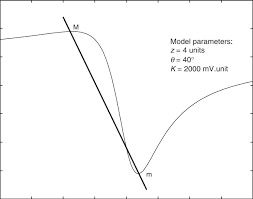The image above represents gravity anomaly for an infinitely long cylinder.

To compute for gravity anomaly for an infinitely long cylinder, five essential parameters are needed and these parameters are Gravitational Constant (G), Anomalous Density (Δρ), Radius of Cylinder (b), Depth Buried (d) and Horizontal Distance (x).

The formula for calculating gravity anomaly for an infinitely long cylinder:

δgx = GΔρ2πb²d / x² + d²

Where:

δgx = Gravity Anomaly for an Infinitely Long Cylinder
G = Gravitational Constant
Δρ = Anomalous Density
d = Depth Buried
x = Horizontal Distance

Let’s solve an example;
Find the gravity anomaly for an infinitely long cylinder when the gravitational constant is 6.67E-11, the anomalous density is 9, the radius of cylinder is 11, the depth buried is 8 and the horizontal distance is 10.

This implies that;

G = Gravitational Constant = 6.67E-11
Δρ = Anomalous Density = 9
b = Radius of Cylinder = 11
d = Depth Buried = 8
x = Horizontal Distance = 10

δgx = GΔρ2πb²d / x² + d²
δgx = 6.67e-11(9)2π(11)²(8) / (10)² + (8)²
δgx = 6.67e-11(9)2π(121)(8) / (100) + (64)
δgx = 6.67e-11(9)(6082.123) / 164
δgx = 0.000003651098 / 164
δgx = 2.226e-8

Therefore, the gravity anomaly for an infinitely long cylinder is 2.226e-8 mGal.

Nickzom Calculator – The Calculator Encyclopedia is capable of calculating the gravity anomaly for an infinitely long cylinder.

To get the answer and workings of the gravity anomaly for an infinitely long cylinder using the Nickzom Calculator – The Calculator Encyclopedia. First, you need to obtain the app.

You can get this app via any of these means:

To get access to the professional version via web, you need to register and subscribe for NGN 2,000 per annum to have utter access to all functionalities.
You can also try the demo version via https://www.nickzom.org/calculator

Apple (Paid) – https://itunes.apple.com/us/app/nickzom-calculator/id1331162702?mt=8
Once, you have obtained the calculator encyclopedia app, proceed to the Calculator Map, then click on Geology under Add-on.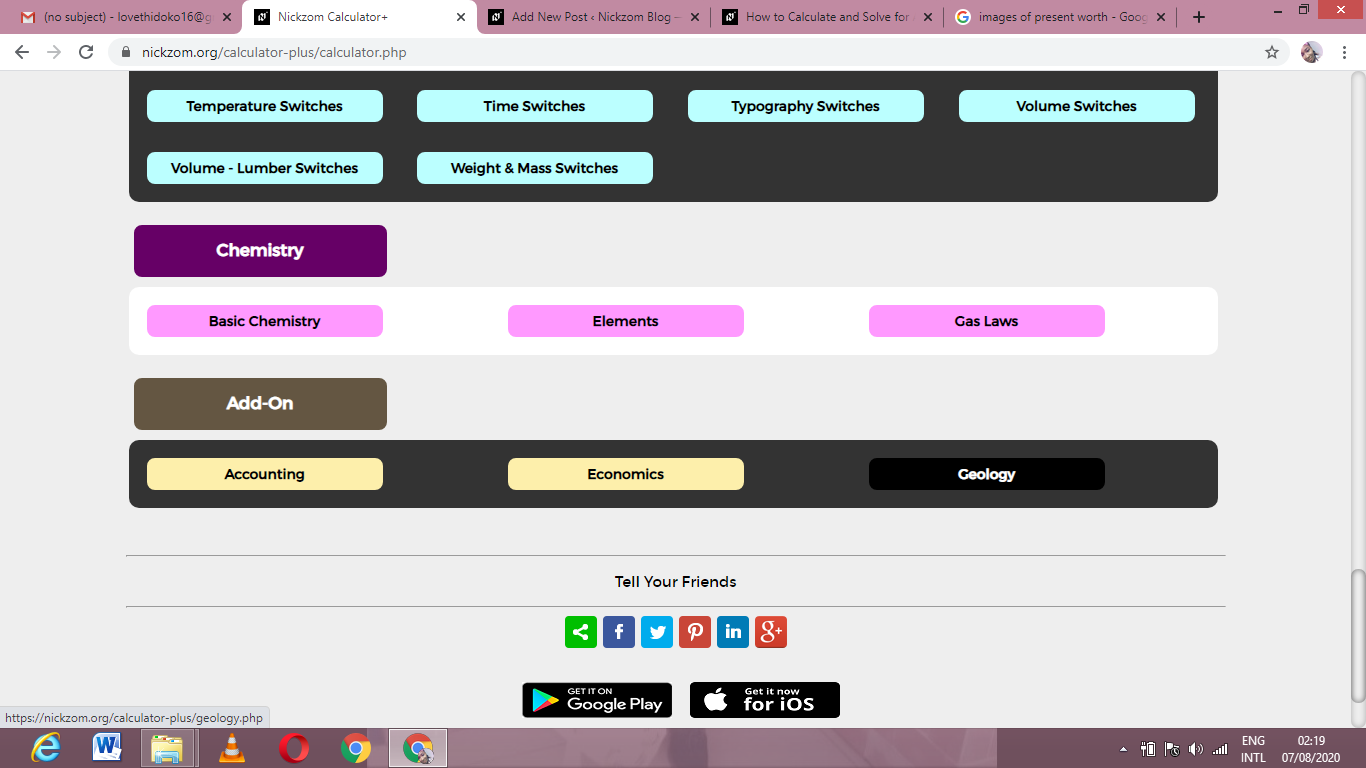Now, Click on Gravity under Geology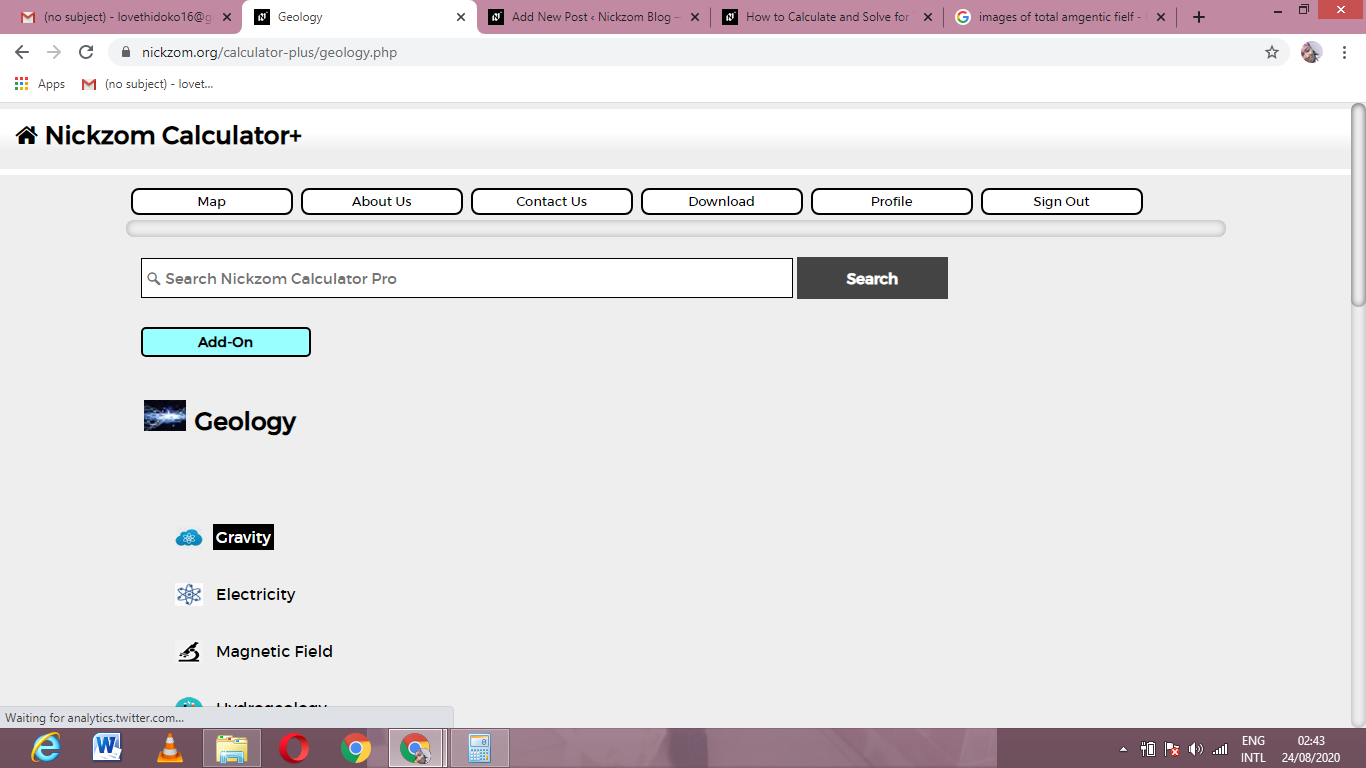Now, Click on Gravity Anomaly for an Infinitely Long Cylinder under Gravity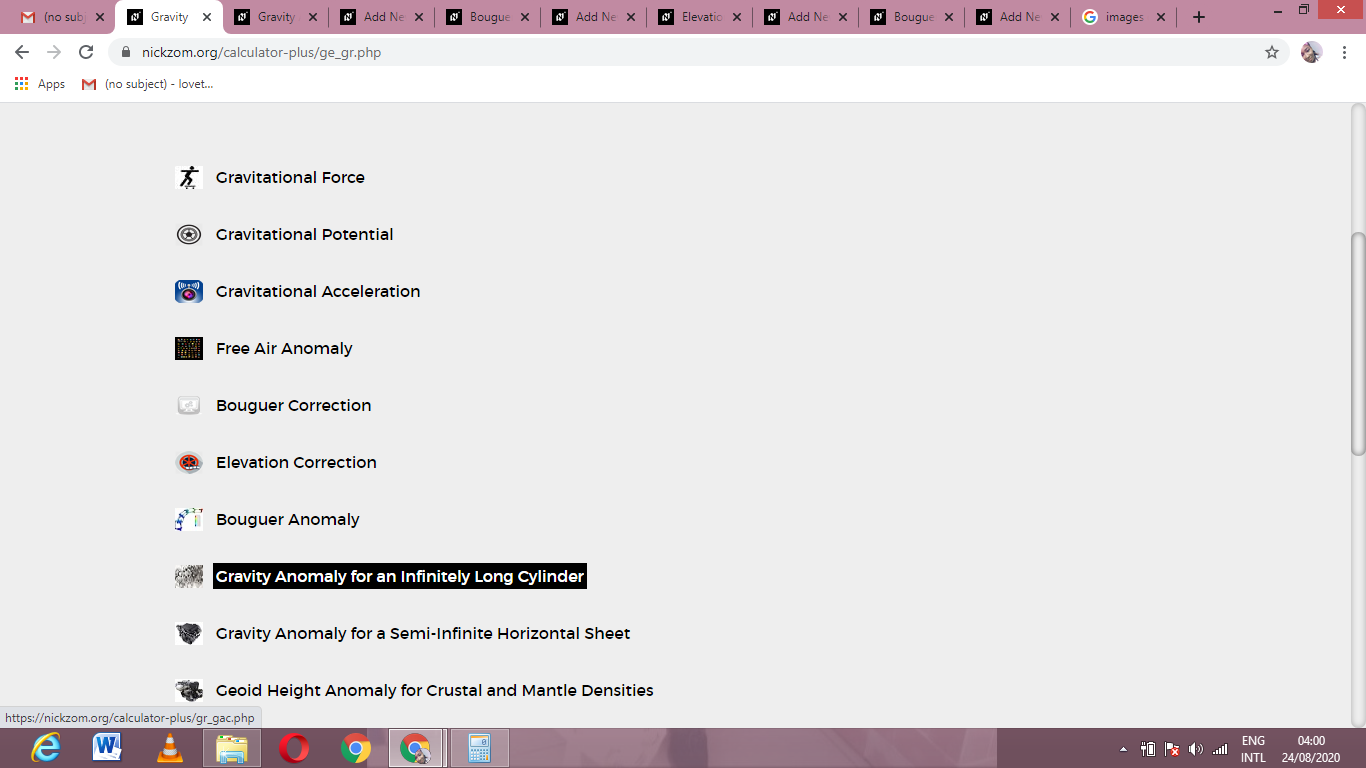The screenshot below displays the page or activity to enter your values, to get the answer for the gravity anomaly for an infinitely long cylinder according to the respective parameters which are the Gravitational Constant (G), Anomalous Density (Δρ), Radius of Cylinder (b), Depth Buried (d) and Horizontal Distance (x).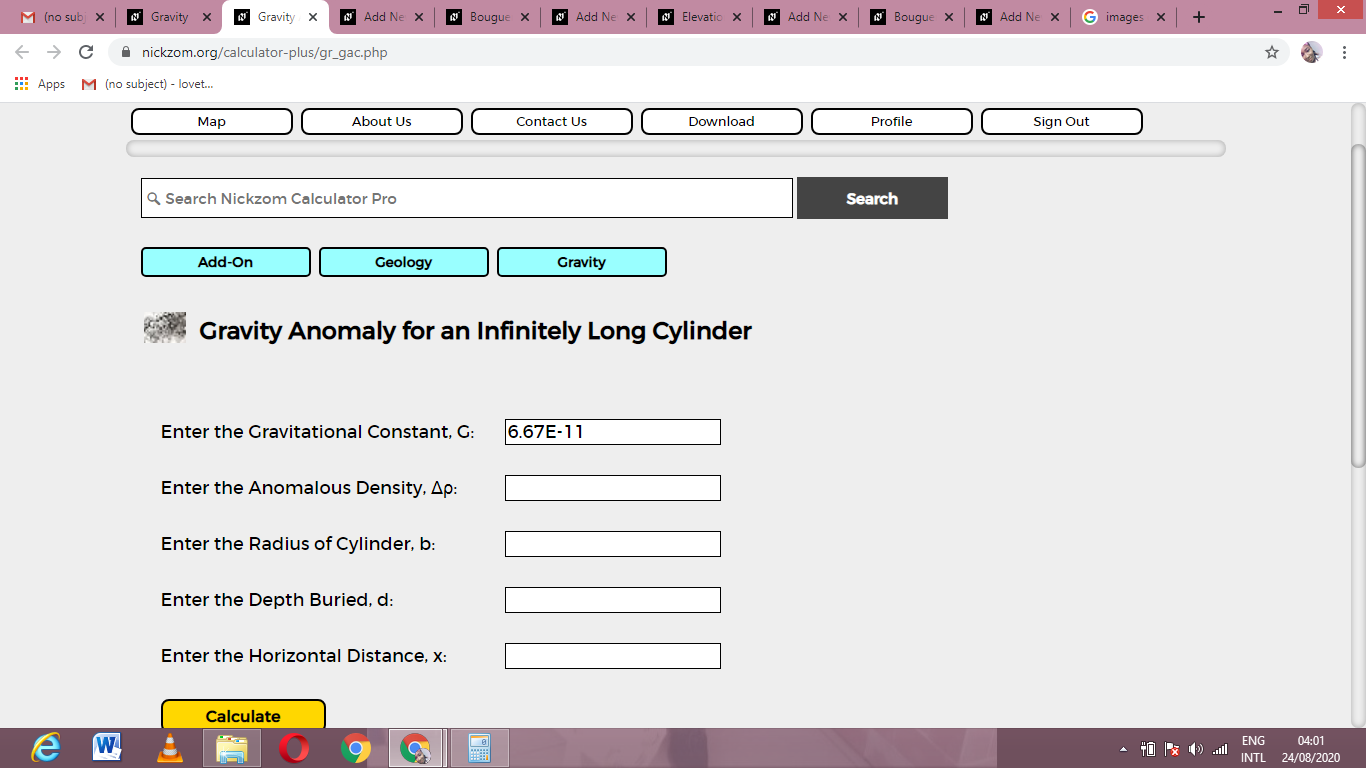Now, enter the values appropriately and accordingly for the parameters as required by the Gravitational Constant (G) is 6.67E-11, Anomalous Density (Δρ) is 9, Radius of Cylinder (b) is 11, Depth Buried (d) is 8 and Horizontal Distance (x) is 10.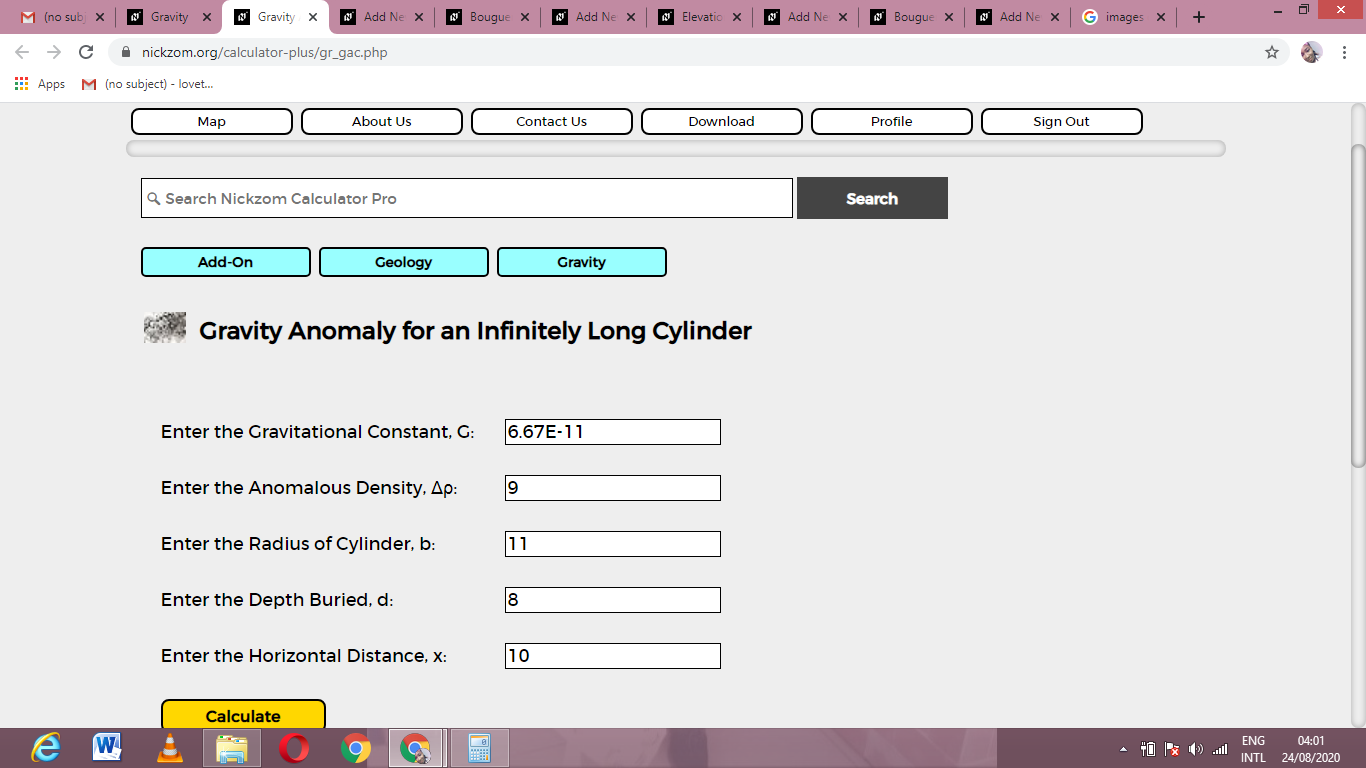Finally, Click on Calculate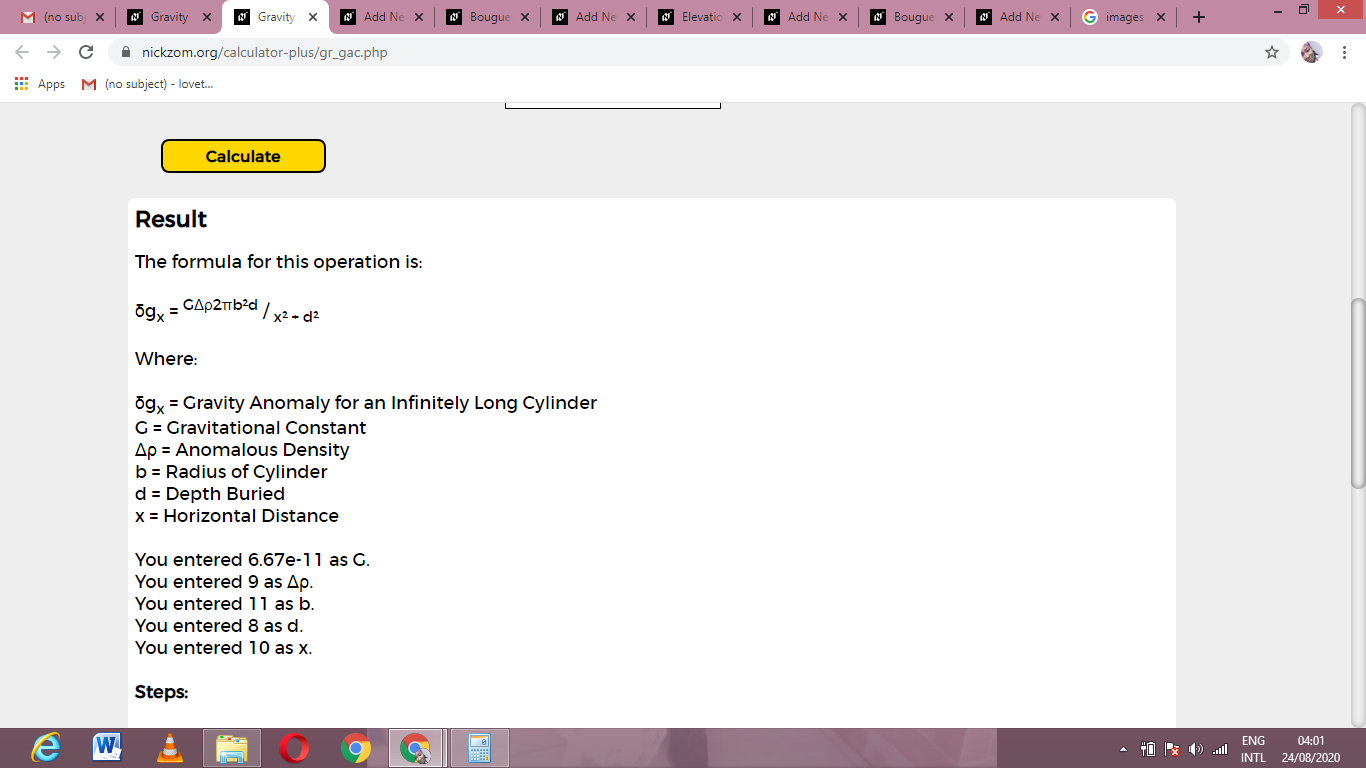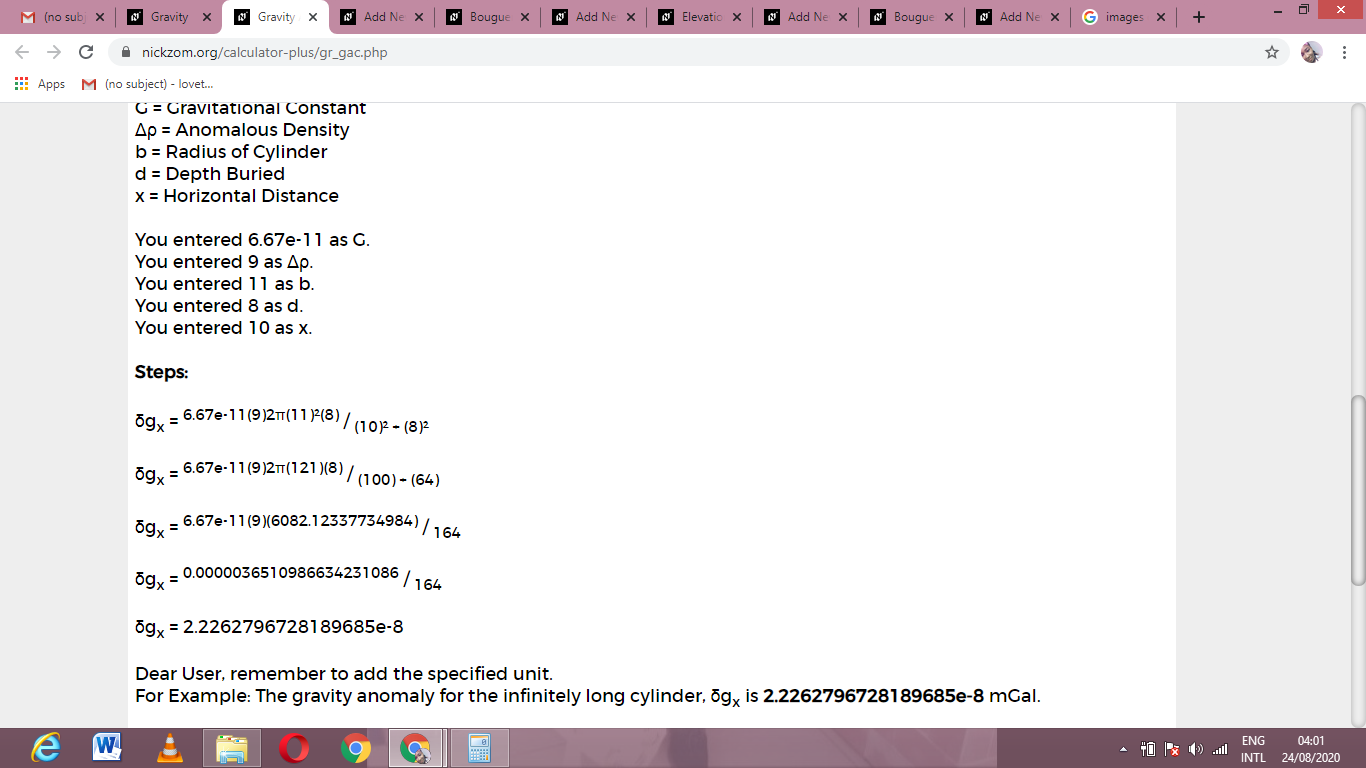As you can see from the screenshot above, Nickzom Calculator– The Calculator Encyclopedia solves for the gravity anomaly for an infinitely long cylinder and presents the formula, workings and steps too.